20.1
19.2
19.1
18.2
18.1
17.2

# Totals

The Grid dashboard item enables you to add a summary value (a total) calculated against displayed values of an individual column, and show the result under this column. Note that you can add any number of totals for each column. For example, you can obtain the number of column records, average or maximum value, etc.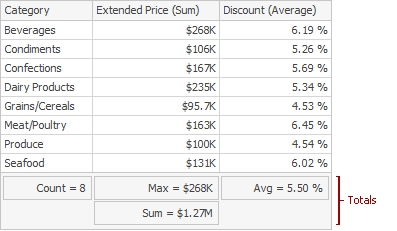This topic describes how to create, edit or clear totals.

The topic consists of the following sections.

## Totals Overview

You can use the following summary functions when creating totals.

 Count The number of records. Sum The sum of the values.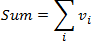Min The smallest value. Max The largest value. Average The average of the values.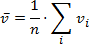Auto The total is calculated using the type of summary function specified for the measure corresponding to the current Grid column. Note that in this case, the total is calculated based on values of the corresponding data field from the underlying data source.

You can create totals using different sets of summary functions. This depends on the type of the data source field providing data for the target column.

Icon Data Source Field Type Supported Totals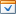Boolean Count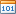Byte Count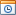Date-time Min, Max, Count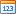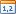Numeric All available types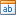String Min, Max, Count
##### IMPORTANT

Note that the Auto type is available only for the Measure column.

## Create and Edit Totals

To create a total, use the context menu of the column header. Right-click the required column header, select Add Total and specify the type of summary function used to calculate a total.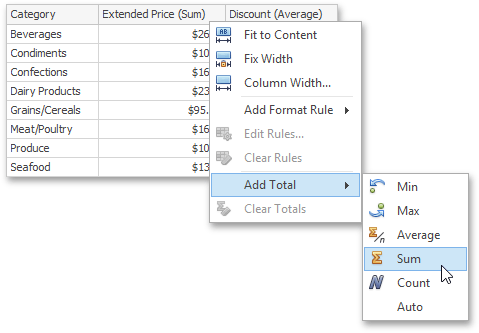To change the total type, right-click the required total and select a new total type.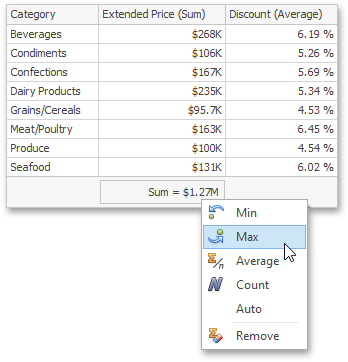## Clear Totals

You can delete one total or all the totals in a particular column.

• To delete a single total, right-click a total and select Remove.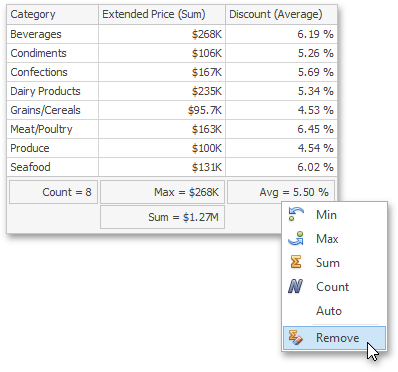• To delete all column totals, right-click the column header and select Clear Totals in the invoked context menu.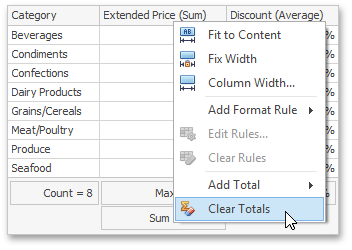## Create Totals in Code

The collection of column totals can be accessed using the GridColumnBase.Totals property.

To create a total for the required column, do the following.

Note that different sets of summary functions can be used to create totals. This depends on the type of the data source field providing data for the target column. The table below lists total types supported by different data source field types.

Data Source Field Type

Supported Totals

Boolean

GridColumnTotalType.Count

Byte

GridColumnTotalType.Count

Date-time

GridColumnTotalType.Min

GridColumnTotalType.Max

GridColumnTotalType.Count

Numeric

All types listed in the GridColumnTotalType enumeration.

String

GridColumnTotalType.Min

GridColumnTotalType.Max

GridColumnTotalType.Count

Totals, except the GridColumnTotalType.Auto type, are calculated based on summary values. When the GridColumnTotal.TotalType property is set to GridColumnTotalType.Auto, the total is calculated based on values of the corresponding data field from the underlying data source. In this case, the Measure.SummaryType property value is used to calculate the total.

The following code sample demonstrates how to add totals to the Measure column.

• The Max total displays the maximum value in the RetailPrice column.
• The Count total displays the number of the column records.
``````using DevExpress.DashboardCommon;

private void Form1_Load(object sender, EventArgs e) {
// Creates a new Grid dashboard item.
GridDashboardItem grid = new GridDashboardItem();

// ...

// Creates a new grid measure column.
GridMeasureColumn colRetailPrice = new GridMeasureColumn(new Measure("RetailPrice"));

// Creates totals of the specified total type.
GridColumnTotal totalMax = new GridColumnTotal() { TotalType = GridColumnTotalType.Max };
GridColumnTotal totalCount = new GridColumnTotal() { TotalType = GridColumnTotalType.Count };

// Adds the created totals to the column's Totals collection.